## Transactions

 Question 1

Consider a schedule of transactions T1 and T2:Here, RX stands for “Read(X)” and WX stands for “Write(X)”. Which one of the following schedules is conflict equivalent to the above schedule?

 AB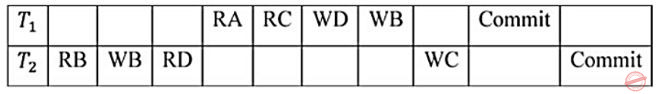CD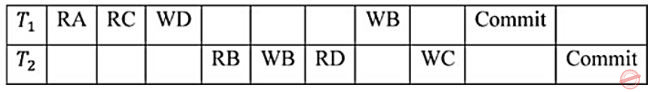Question 1 Explanation:
• Two schedules are said to be conflict equivalent, if conflict operations in both the schedules are executed in the same order.
• First, let’s list the conflict operations of each of the schedule given in the options and compare with the conflict operations of schedule which is given in the question.
Given schedule:Conflict operations:
R2(B) → W1(B)
W2(B) → W1(B)
R1(C) → W2(C)
R2(D) → W1(D)
Option(1):Conflict operations:
R1(C) → W2(C)
W1(D) → R2(D)
W1(B) → R2(B)
W1(B) → W2(B)
Option(2):Conflict operations:
R2(B) → W1(B)
W2(B) → W1(B)
R2(D) → W1(D)
R1(C) → W2(C)
Option(3):Conflict operations:
R2(B) → W1(B)
W2(B) → W1(B)
R2(D) → W1(D)
W2(C) → R1(C)
Option(4):Conflict operations:
R1(C) → W2(C)
W1(D) → R2(D)
R2(B) → W1(B)
W2(B) → W1(B)
The conflict operations in the option (2) and given schedule are appearing in the same sequence order, so option (2) is the answer.
 Question 2
Let Ri(z) and Wi(z) denote read and write operations on a data element z by a transaction Ti, respectively. Consider the schedule S with four transactions.
S: R4(x) R2(x) R3(x) R1(y) W1(y) W2(x) W3(y) R4(y)
Which one of the following serial schedules is conflict equivalent to S
 ABCDQuestion 2 Explanation:
The given schedule ‘s’ is:Precedence GraphT1 → T3 → T4 → T2
 Question 3
For the schedule given below, which of the following is Correct?
3 Write A
5 Write A
6 Write B
8 Write B
 A This schedule is serialized and can occur in a scheme using 2PL protocol B This schedule is serializable but cannot occur in a scheme using 2PL protocol C This schedule is not serialiable but can occur in a scheme using 2PL protocol D This schedule is not seralisable and cannot occur in a scheme using 2PL protocol.
Question 3 Explanation:
Let's draw precedence graph,Since cycle exist so not conflict serializable.
And we know that if the schedule is not serializable then it is not 2PL.
Hence correct option is (D).
 Question 4

Which of the following scenarios may lead to an irrecoverable error in a database system?

 A A transaction writes a data item after it is read by an uncommitted transaction B A transaction reads a data item after it is read by an uncommitted transaction C A transaction reads a data item after it is written by a committed transaction D A transaction reads a data item after it is written by an uncommitted transaction
Question 4 Explanation:
Irrecoverable error occurs when a transaction reads a data item after it is written by uncommitted transaction.
 Question 5

Consider three data items D1, D2 and D3 and the following execution schedule of transactions T1, T2 and T3. In the diagram, R(D) and W(D) denote the actions reading and writing the data item D respectively.Which of the following statements is correct?

 A The schedule is serializable as T2; T3; T1 B The schedule is serializable as T2; T1; T3 C The schedule is serializable as T3; T2; T1 D The schedule is not serializable
Question 5 Explanation:
Precedence graph:Cycle formed so not serializable.
 Question 6

Consider the following log sequence of two transactions on a bank account, with initial balance 12000, that transfer 2000 to a mortgage payment and then apply a 5% interest.

```
1. T1 start
2. T1 B old=12000 new=10000
3. T1 M old=0 new=2000
4. T1 commit
5. T2 start
6. T2 B old=10000 new=10500
7. T2 commit
```

Suppose the database system crashes just before log record 7 is written. When the system is restarted, which one statement is true of the recovery procedure?

 A We must redo log record 6 to set B to 10500. B We must undo log record 6 to set B to 10000 and then redo log records 2 and 3. C We need not redo log records 2 and 3 because transaction T1 has committed. D We can apply redo and undo operations in arbitrary order because they are idempotent.
Question 6 Explanation:
When the database system crashes after the transactions have committed then we need to redo the log records. And if the database system crashes before the transactions have committed then we need to undo the log records.
So from above theory we can say that option (B) is the correct answer.
 Question 7
Let S be the following schedule of operations of three transactions T1, T2 and T3in a relational database system:
R2(Y), R1(X), R3(Z), R1(Y), W1(X), R2(Z), W2(Y), R3(X), W3(Z)
Consider the statements P and Q below:
P: S is conflict-serializable.
Q: If T3commits before T1finishes, then S is recoverable.
Which one of the following choices is correct?
 A P is true and Q is false. B Both P and Q are false. C Both P and Q are true. D P is false and Q is true.
Question 7 Explanation:Question 8
Let ri(z) and wi(z) denote read and write operations respectively on a data item z by a transaction Ti. Consider the following two schedules.
S1: r1(x) r1(y) r2(x) r2(y) w2(y) w1(x)
S2: r1(x) r2(x) r2(y) w2(y) r1(y) w1(x)
Which one of the following options is correct?
 A S1is not conflict serializable, and S2 is conflict serializable. B Neither S1nor S2is conflict serializable. C Both S1and S2are conflict serializable. D S1is conflict serializable, and S2is not conflict serializable.
Question 8 Explanation:Question 9

Consider the following two transactions: T1 and T2.

```T1: read(A);
if A=0 then B ← B+1;
write(B);
if B≠0 then A ← A-1;
write(A);
Which of the following schemes, using shared and exclusive locks, satisfy the requirements for strict two phase locking for the above transactions?
```
 ABC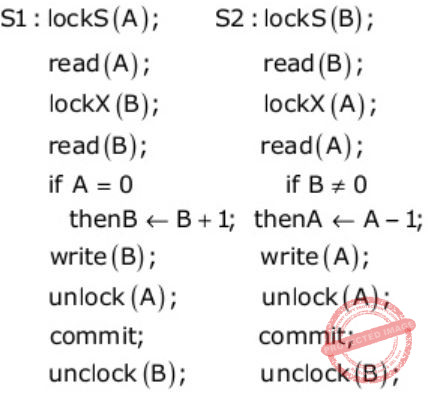DQuestion 9 Explanation:
For strict 2PL the requirements are,
i) Exclusive locks should be released after the commit.
ii) No locking can be done after the first unlock.
(A) Wrong because to write x you need Exclusive Lock.
(B) Wrong because violating the 1st requirement.
(C) Correct.
(D) Wrong because violating the 1st requirement.
 Question 10

Consider the following three schedules of transactions T1, T2 and T3. [Notation: In the following NYO represents the action Y (R for read, W for write) performed by transac­tion N on object O.]

```(S1)	2RA	2WA	3RC	2WB	3WA	3WC	1RA	1RB	1WA	1WB
(S2)	3RC	2RA	2WA	2WB	3WA	1RA	1RB	1WA	1WB	3WC
(S3)	2RA	3RC	3WA	2WA	2WB	3WC	1RA	1RB	1WA	1WB```
Which of the following statements is TRUE?
 A S1, S2 and S3 are all conflict equivalent to each other B No two of S1, S2 and S3 are conflict equivalent to each other C S2 is conflict equivalent to S3, but not to S1 D S1 is conflict equivalent to S2, but not to S3
Question 10 Explanation:
Two schedules are conflict equivalent when the precedence graph are isomorphic.
For S1:For S2:For S3: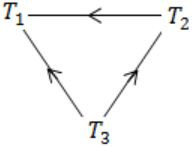Hence, S1 is conflict equivalent to S2, but not to S3.
 Question 11

Consider the following schedules involving two transactions. Which one of the following statements is TRUE?

S1: r1(X); r1(Y); r2(X); r2(Y); w2(Y); w1(X)
S2: r1(X); r2(X); r2(Y); w2(Y); r1(Y); w1(X)
 A Both S1 and S2 are conflict serializable. B S1 is conflict serializable and S2 is not conflict serializable. C S1 is not conflict serializable and S2 is conflict serializable. D Both S1 and S2 are not conflict serializable.
Question 11 Explanation:In precedence graph of S1 since cycle is formed so not conflict serializable.
But in precedence graph of S2 No cycle is formed so it is conflict serializable.
 Question 12

Consider the following transaction involving two bank account x and y.

The constraint that the sum of the accounts x and y should remain constant is that of

 A Atomicity B Consistency C Isolation D Durability
Question 12 Explanation:
The consistency property ensures that the database remains in a consistent state before the (start of the transaction and after the transaction is over. Here sum of the accounts x & y should remain same before & after execution of the given transactions which refers to the consistency of the sum.
 Question 13

Consider a simple checkpointing protocol and the following set of operations in the log.

(start, T4); (write, T4, y, 2, 3); (start, T1); (commit, T4); (write, T1, z, 5, 7);
(checkpoint);
(start, T2); (write, T2, x, 1, 9); (commit, T2); (start, T3); (write, T3, z, 7, 2);

If a crash happens now and the system tries to recover using both undo and redo operations, what are the contents of the undo list and the redo list

 A Undo T3, T1; Redo T2 B Undo T3, T1; Redo T2, T4 C Undo: none; redo: T2, T4, T3, T1 D Undo T3, T1; T4; Redo: T2
Question 13 Explanation:
As T1 & T3 are not yet committed they must be undone. The transactions which are after the latest checkpoint must be redone. So T2 must be redone. No need to redo the records which are before the last checkpoint, so T4 need not be redone.
 Question 14

Suppose a database schedule S involves transactions T1, ..., Tn. Construct the precedence graph of S with vertices representing the transactions and edges representing the conﬂicts. If S is serializable, which one of the following orderings of the vertices of the precedence graph is guaranteed to yield a serial schedule?

 A Topological order B Depth-ﬁrst order C Breadth-ﬁrst order D Ascending order of transaction indices
Question 14 Explanation:
If a schedule is conflict serializable then no cycle in precedence graph should be present.
But BFS and DFS are also possible for cyclic graphs.
And topological sort is not possible for cyclic graph.
Moreover option (D) is also wrong because in a transaction with more indices might come before lower one.
 Question 15
Consider the following database schedule with two transactions, T1 and T2.
S = r2(X); r1(X); r2(Y); w1(X); r1(Y); w2(X); a1; a2
where ri(Z) denotes a read operation by transaction Ti on a variable Z, wi(Z) denotes a write operation by Ti on a variable Z and ai denotes an abort by transaction Ti.
Which one of the following statements about the above schedule is TRUE?
 A S is non-recoverable B S is recoverable, but has a cascading abort C S does not have a cascading abort D S is strict
Question 15 Explanation:
Given schedule is,Now let's check statements one by one,
A) False, because there is no dirty read. So, it is recoverable.
B) False, because there is to dirty read. So, no cascading aborts.
C) True.
D) False, because there is Transaction T2 which written the value of x which is written by T1 before T1 has aborted. So, not strict.
 Question 16
Which of the following is NOT a part of the ACID properties of database transactions?
 A Atomicity B Consistency C Isolation D Deadlock-freedom
Question 16 Explanation:
A transaction in a database system must maintain Atomicity, Consistency, Isolation and Durability – commonly known as ACID properties.
So, Deadlock – freedom is not there in the ACID properties.
 Question 17
Two transactions T1 and T2 are given as
T1: r1(X)w1(X)r1(Y)w1(Y)
T2: r2(Y)w2(Y)r2(Z)w2(Z)
where ri(V) denotes a read operation by transaction Ti on a variable V and wi(V) denotes a write operation by transaction Ti on a variable V. The total number of conflict serializable schedules that can be formed by T1 and T2 is ____________.
 A 54 B 55 C 56 D 57
Question 17 Explanation:
From the given transactions T1 and T2, total number of schedules possible = (4+4)!/4!4!
= 8!/(4×3×2×4×3×2)
= (8×7×6×5×4×3×2×1)/(4×3×2×4×3×2)
= 70
Following two conflict actions are possible: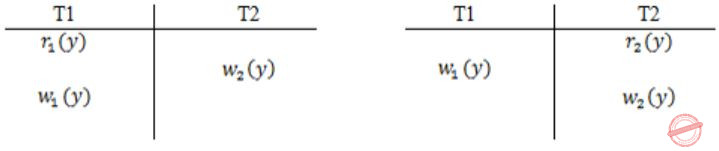∴# Permutations = 4 × 3 = 12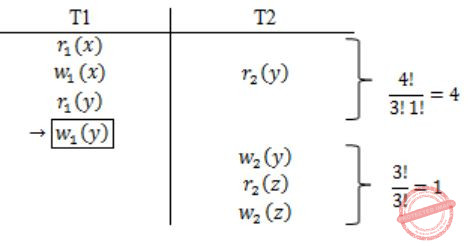#permutations = 4 × 1 = 4
∴ Total no. of conflict serial schedules possible = 70 - 12 - 4 = 54
 Question 18
Consider the following two statements about database transaction schedules:
I. Strict two-phase locking protocol generates conflict serializable schedules that are also recoverable.
II. Timestamp-ordering concurrency control protocol with Thomas Write Rule can generate view serializable schedules that are not conflict serializable.
Which of the above statements is/are TRUE?
 A Both I and II B Neither I nor II C II only D I only
Question 18 Explanation:
(Memory-based question)
In strict 2PL, a transaction T does not release any of its exclusive (write) locks until after it commits or aborts.
Hence, no other transaction can read or write an item that is written by T unless T has committed, leading to a strict schedule for recoverability.
(Ref: Fundamentals of Database Systems by Elmasri and Navathe, 7e Pg. No. 789)
By ignoring the write, Thomas write rule allows schedules that are not conflict serializable but are nevertheless correct.
Those non-conflict-serializable schedules allowed satisfy the definition of view serializable schedules.
(Ref: Database System Concepts by Silberschatch, Korth and Sudarshan, 6e Pg No. 686)
 Question 19

Consider the following partial Schedule S involving two transactions T1 and T2. Only the read and the write operations have been shown. The read operation on data item P is denoted by read(P) and the write operation on data item P is denoted by write(P).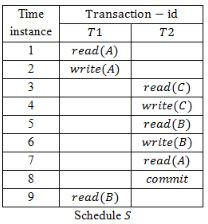A T2 must be aborted and then both T1 and T2 must be re-started to ensure transaction atomicity B Schedule S is non-recoverable and cannot ensure transaction atomicity C Only T2 must be aborted and then re-started to ensure transaction atomicity D Schedule S is recoverable and can ensure atomicity and nothing else needs to be done
Question 19 Explanation:
T2 is reading the value written by T1 and getting committed before T1 commits. So it is non-recoverable schedule.
 Question 20

Consider the following four schedules due to three transactions (indicated by the subscript) using read and write on a data item x, denoted by r(x) and w(x) respectively. Which one of them is conflict serializable?

 A r1 (x); r2 (x); w1 (x); r3 (x); w2 (x) B r2 (x);r1 (x);w2 (x);r3 (x);w1 (x) C r3 (x);r2 (x);r1 (x);w2 (x);w1 (x) D r2 (x);w2 (x);r3 (x);r1 (x);w1 (x)
Question 20 Explanation:
Option: A- Polygraph contains cycle. So, not a conflict serializable.
Option: B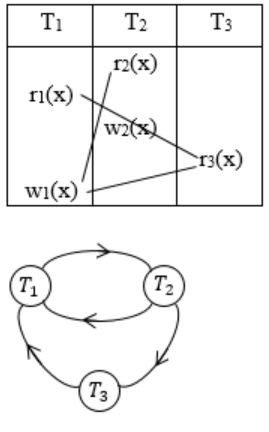-Cyclic
Option: C- Cyclic
Option: D- Acyclic, so conflict serializable.
 Question 21

Consider two transactions T1 and T2, and four schedules S1, S2, S3, S4 of T1 and T2 as given below:

T1 = R1[X] W1[X] W1[Y]
T2 = R2[X] R2[Y] W2[Y]
S1 = R1[X] R2[X] R2[Y] W1[X] W1[Y] W2[Y]
S2 = R1[X] R2[X] R2[Y] W1[X] W2[Y] W1[Y]
S3 = R1[X] W1[X] R2[X] W1[Y] R2[Y] W2[Y]
S1 = R1[X] R2[Y]R2[X]W1[X] W1[Y] W2[Y]

Which of the above schedules are conflict-serializable?

 A S1 and S2 B S2 and S3 C S3 only D S4 only
Question 21 Explanation:
S1 has a cycle from T1→T2 and T2→T1 Schedule S2,Dependency graph is,So, there is no cycle.
Schedule S3,Dependency graph is,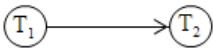S4 also has a cycle T1→T2 and T2→T1.
So, S2 and S3 are conflict serializable.
There are 21 questions to complete.

Register Now